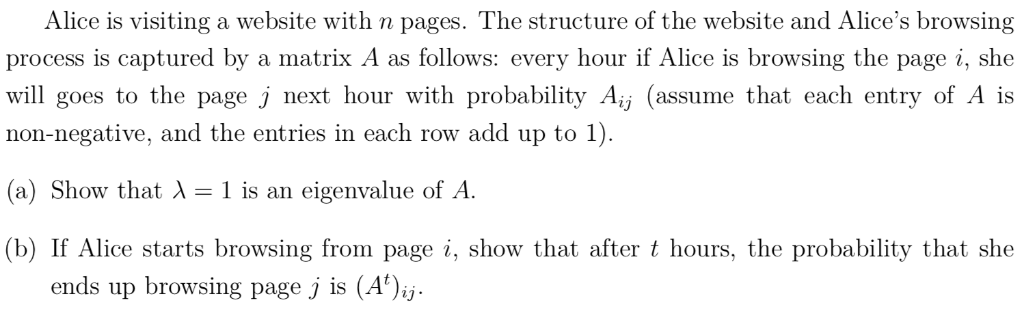# Alice is visiting a website with n pages. The structure of the website and Alice's browsing process is captured by...Alice is visiting a website with n pages. The structure of the website and Alice's browsing process is captured by a matrix A as follows: every hour if Alice is browsing the page i, she will goes to the page j next hour with probability Aj (assume that each entry of A is non-negative, and the entries in each row add up to 1) (a) Show that λ is an eigenvalue of A. (b) If Alice starts browsing from page i, show that after t hours, the probability that she ends up browsing page j is (A )ij.

a) Suppose Alice starts with page; then, in the next hour she will be at exactly one of thepages,with probabilitiesrespectively. Thus, the probability that Alice will be at any one of thepages,isBut this probability isbecause in the next hour she will definitely be at one of thepages; this meansand this is true for all. LetThenfor all. This means. Thus,is an eigenvalue of.

b) We use induction on. Ifthen by definition the probability that Alice ends up browsing page(starting from page) afterhours is. Thus, the statement in question is true for.

Suppose that the statement is true for some. Now suppose that Alice starts at page. Suppose that afterhours she is at page. Then the probability that she will be at pageinhour is (by base case and induction hypothesis)Sincecan be any of thepages, the probability that she is at pageinhour isThus, the statement in question is true for. By induction, the statement is true for all​​​​​​​.

##### Add Answer of: Alice is visiting a website with n pages. The structure of the website and Alice's browsing process is captured by...
More Homework Help Questions Additional questions in this topic.